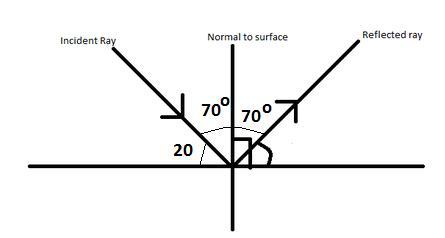# A ray of light falls on a plane mirror forming an angle of 20 degree with the mirror. The deviation of the rayГўв‚¬вЂ№ on reflection is?

Given: A ray of light falls on a plane mirror forming an angle of 20 degree with the mirror.

To find: The deviation of the ray​ on reflection

Solution:

The angle between the normal and the plane of the mirror is 90 degree.

So, the angle between the incident ray and the normal will be 90 - 20 = 70 degree.Formula for the angle of deviation is $180 - 2i$ degree

So, the angle of deviation = $180 - 2\times70$

= 40 degree

Updated on: 10-Oct-2022

539 Views